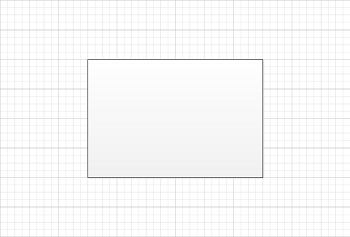# Calculate Pin Values and Setting Size of a Shape

Contents
[ ]

## Calculate PinX and PinY Values of the Sub Shape

If the shape is a children of group shape, it’s xform is a relative coordinate of it’s parent shape，but not absolute coordinate in the Page. If the user require to get the absolute coordinate, then this sample code helps.

A point specified in local coordinates can be converted into parent coordinates by applying the following transformations in the following order:

1. Subtract the value of the LocPinX property of the Cell_Type element from the x-coordinate.
2. Subtract the value of the LocPinY property of the Cell_Type from the y-coordinate.
3. Mirror the point about the y-axis if the value of the FlipX property of the Cell_Type is equal to one.
4. Mirror the point about the x-axis if the value of the FlipY property of the Cell_Type is equal to one.
5. Rotate the point counterclockwise around the origin by the value of the Angle property of the Cell_Type.
6. Add the value of the PinX Cell_Type to the x-coordinate.
7. Add the value of the PinY Cell_Type to the y-coordinate.

### Calculate PinX and PinY Programming Sample

Use the following code in your Java application to calculate PinX and PinY values of a sub-shape using Aspose.Diagram for Java API.

## Setting Height and Width of a Shape

The Shape Class allows you to control the shape size by specifying height and width of the shape using SetHeight and SetWidth methods.

The SetHeight and SetWidth methods, exposed by the Shape class, support resizing a shape with the master, without the master or in the form of a group shape.

The code examples in this article set the Height and Width to resize the shape on the page.

Input diagramThe diagram after the Height and Width have been changedThe process for setting Height and Width is: